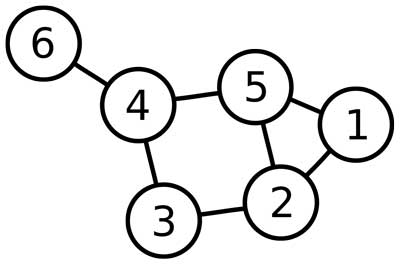# The Graph data structure

## #data-structures series

Posted on February 15, 2016

The #data-structures series is a collection of posts about reimplemented data structures in JavaScript.

If you are not familiar with data structures, a quick introduction and the full list of reimplemented data structures can be found in the introduction post of the series on data structures in JavaScript.

If you feel comfortable with the concept of each data structure and only want to see the code, have a look at the summary post of the series. It removes all explanations and contains only the JavaScript code for all data structures discussed in the series.

## Get the code on Github

Of course, all the code can also be found on Github in the repository data-structures-in-javascript.

# The Graph data structure

## Definition

A Graph data structure consists of a finite (and possibly mutable) set of vertices or nodes or points, together with a set of unordered pairs of these vertices for an undirected Graph or a set of ordered pairs for a directed Graph. These pairs are known as edges, arcs, or lines for an undirected Graph and as arrows, directed edges, directed arcs, or directed lines for a directed Graph. The vertices may be part of the Graph structure, or may be external entities represented by integer indices or references. From Wikipedia

A Graph data structure may also associate to each edge some edge value, such as a symbolic label or a numeric attribute (cost, capacity, length, etc.).

## Representation

There are different ways of representing a graph, each of them with its own advantages and disadvantages. Here are the main 2:

• Adjacency list: For every vertex a list of adjacent vertices is stored. This can be viewed as storing the list of edges. This data structure allows the storage of additional data on the vertices and edges.
• Adjacency matrix: Data are stored in a two-dimensional matrix, in which the rows represent source vertices and columns represent destination vertices. The data on the edges and vertices must be stored externally.

## Complexity

O(|V|+|E|) O(1) O(1) O(|V|)
O(|V|^2) O(|V|^2) O(1) O(1)

To get a full overview of the time and space complexity of the Graph data structure, have a look to this excellent Big O cheat sheet.

## Our sample graph

The following sample graph is the one that our code sample will reproduce. It is a nice image from wikipedia on the Graph theory.## The code

The code below uses the adjacency list representation.

``````function Graph() {
this.vertices = [];
this.edges = [];
this.numberOfEdges = 0;
}

this.vertices.push(vertex);
this.edges[vertex] = [];
};
Graph.prototype.removeVertex = function(vertex) {
var index = this.vertices.indexOf(vertex);
if(~index) {
this.vertices.splice(index, 1);
}
while(this.edges[vertex].length) {
}
};
this.edges[vertex1].push(vertex2);
this.edges[vertex2].push(vertex1);
this.numberOfEdges++;
};
Graph.prototype.removeEdge = function(vertex1, vertex2) {
var index1 = this.edges[vertex1] ? this.edges[vertex1].indexOf(vertex2) : -1;
var index2 = this.edges[vertex2] ? this.edges[vertex2].indexOf(vertex1) : -1;
if(~index1) {
this.edges[vertex1].splice(index1, 1);
this.numberOfEdges--;
}
if(~index2) {
this.edges[vertex2].splice(index2, 1);
}
};
Graph.prototype.size = function() {
return this.vertices.length;
};
Graph.prototype.relations = function() {
return this.numberOfEdges;
};
Graph.prototype.traverseDFS = function(vertex, fn) {
if(!~this.vertices.indexOf(vertex)) {
}
var visited = [];
this._traverseDFS(vertex, visited, fn);
};
Graph.prototype._traverseDFS = function(vertex, visited, fn) {
visited[vertex] = true;
if(this.edges[vertex] !== undefined) {
fn(vertex);
}
for(var i = 0; i < this.edges[vertex].length; i++) {
if(!visited[this.edges[vertex][i]]) {
this._traverseDFS(this.edges[vertex][i], visited, fn);
}
}
};
Graph.prototype.traverseBFS = function(vertex, fn) {
if(!~this.vertices.indexOf(vertex)) {
}
var queue = [];
queue.push(vertex);
var visited = [];
visited[vertex] = true;

while(queue.length) {
vertex = queue.shift();
fn(vertex);
for(var i = 0; i < this.edges[vertex].length; i++) {
if(!visited[this.edges[vertex][i]]) {
visited[this.edges[vertex][i]] = true;
queue.push(this.edges[vertex][i]);
}
}
}
};
Graph.prototype.pathFromTo = function(vertexSource, vertexDestination) {
if(!~this.vertices.indexOf(vertexSource)) {
}
var queue = [];
queue.push(vertexSource);
var visited = [];
visited[vertexSource] = true;
var paths = [];

while(queue.length) {
var vertex = queue.shift();
for(var i = 0; i < this.edges[vertex].length; i++) {
if(!visited[this.edges[vertex][i]]) {
visited[this.edges[vertex][i]] = true;
queue.push(this.edges[vertex][i]);
// save paths between vertices
paths[this.edges[vertex][i]] = vertex;
}
}
}
if(!visited[vertexDestination]) {
return undefined;
}

var path = [];
for(var j = vertexDestination; j != vertexSource; j = paths[j]) {
path.push(j);
}
path.push(j);
return path.reverse().join('-');
};
Graph.prototype.print = function() {
console.log(this.vertices.map(function(vertex) {
return (vertex + ' -> ' + this.edges[vertex].join(', ')).trim();
}, this).join(' | '));
};

var graph = new Graph();
graph.print(); // 1 -> | 2 -> | 3 -> | 4 -> | 5 -> | 6 ->
graph.print(); // 1 -> 2, 5 | 2 -> 1, 3, 5 | 3 -> 2, 4 | 4 -> 3, 5, 6 | 5 -> 1, 2, 4 | 6 -> 4
console.log('graph size (number of vertices):', graph.size()); // => 6
console.log('graph relations (number of edges):', graph.relations()); // => 7
graph.traverseDFS(1, function(vertex) { console.log(vertex); }); // => 1 2 3 4 5 6
console.log('---');
graph.traverseBFS(1, function(vertex) { console.log(vertex); }); // => 1 2 5 3 4 6
console.log('path from 6 to 1:', graph.pathFromTo(6, 1)); // => 6-4-5-1
console.log('path from 3 to 5:', graph.pathFromTo(3, 5)); // => 3-2-5
graph.removeEdge(1, 2);
graph.removeEdge(4, 5);
graph.removeEdge(10, 11);
console.log('graph relations (number of edges):', graph.relations()); // => 5
console.log('path from 6 to 1:', graph.pathFromTo(6, 1)); // => 6-4-3-2-5-1